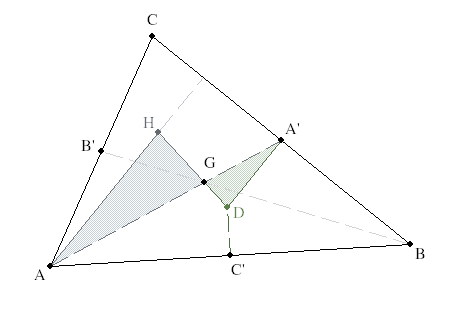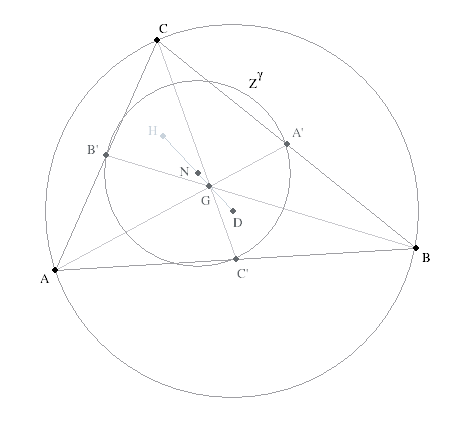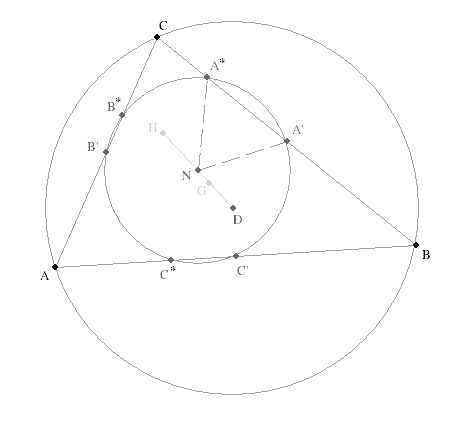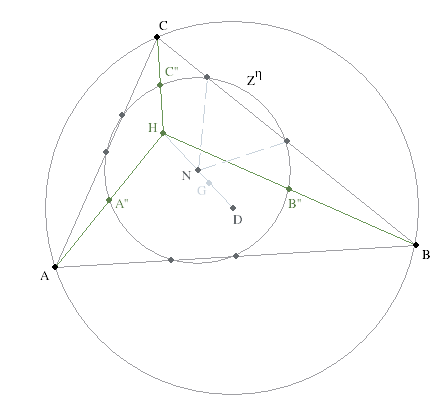## 1. Introduction

In this lesson we introduce a new concept, dilatation, which is an example of a transformation of the Euclidean plane to itself that takes points to point and lines to lines, and has additional, useful properties. Dilatations simplify classical Euclidean proofs and they are an introduction to the fourth and final part of the course where we discover and classify all Euclidean isometries in the plane.

In this lesson we will also pay close attention to the method of proof we apply in any given situation. We begin with Court’s proof of the existence and properties of the Euler line. This proof is in the classical style of Euclid himself, in that it uses the Side-Angle-Side (SAS) theorem for similar triangles you learned in high school.

Then we introduce dilatations but we do not study them systematically and exhaustively (see Tondeur, Ch. 2) because this would distract us from the purpose of this lesson.

We then give a second proof of the same theorem concerning Euler’s line, using dilatation. Finally, we prove a much more complicated theorem about a circle that connects 9 interesting points of any triangle, again using dilatations.

## 2. Court’s Proof of the Euler Line Theorem (ELT)

Recall how we proved the concurrency of the medians of a triangle using vector methods earlier in the course. The trick was to rewrite the barycenter, $G=\frac{A+B+C}{3}$ of a triangle $\triangle ABC$ in three different ways, beginning with $G = \frac{1}{3}A + \frac{2}{3}(\frac{B+C}{2})$ and cycling the three letters. Recall from the Main Collinearity Lemma (MCL), this expresses $G$ as a point $\frac{2}{3}$ of the way down the cevian from vertex $A$ to the midpoint of the opposite side. Cycling the labels puts $G$ on each of the three medians, hence they must intersect. (We even say that the medians of a triangle trisect each other to remind you of this proof.)

Question 1.

Give another proof directly from Ceva’s theorem.

Since the midpoints $A', B', C'$ of the sides have the ratios $\frac{A'-B}{A'-C}= -1$, etc., their product is $-1$. So these cevians are concurrent.

The foregoing discussion used the vector methods we identified with Cartesian geometry in the first part of the course. We next take a more classical, Euclidean approach.

Perhaps you remember from high school that the perpendicular bisectors of the sides of a triangle are also concurrent (as are the altitudes, and the angle bisectors.) We give a different kind of proof for the first and the second concurrency, and skip the angle bisectors.

• For convenience, we will abbreviate the term "perpendicular bisector" to perbis, and write $perbis(AB)$ for the perbis of the segment between $A, B$.

Since for Euclid, a circle is the locus of points equidistant from its center, and the $perbis(AB)$ is the locus of points equidistant from $A \and B$, every circle through A and B must have its center on $c := perbis(AB)$. We use a colon in front of an = sign when we want to emphasize that the equation is a definition, not a deduction.

Let $b=perbis(CA) \and a = perbis(BC)$. Then $(cb)$ is the center of a circle through $A, B \and C$ and so lies on $a$. In other words, $D := (abc)$ exists and is called the circumcenter because it is the center of the circumcircle, $\odot ABC$.

The point were the three altitudes of a triangle meet is called the orthocenter, and usually denoted by the letter $H$. There direct proofs that the orthocenter exists. But we shall derive this fact from the properties of $G \and D$ using, first, a purely Euclidean proof due to Court, and then another proof using dilatations. In the process we identify $H = 3G - 2D$.

Question 2.

How does this equation say that $(HGD)$ and that $G$ trisects the segment $HD$ ?

Since $3 + (-2) =1$ the three points are collinear, and $\frac{H-G}{H-D}= \frac{2}{3}$

So then b and c intersect at the center of the circle D through C and A, and through A and B. This is the circumcircle of the triangle. Since the circumcircle also passes through B and C, its center is on the perbis a of BC. So a,b,c are concurrent.

Exercise 1a.

Use KSEG to draw G,H,D of a triangle. Show that the three points are collinear on the so-called Euler Line, and discover the proportion of H,G, and D on the line. What happens when the triangle becomes obtuse?

Exercise 1b.

Once you have two nice KSEG examples, one for an acute and the other for an obtuse triangle, draw the three points and their line with your gnomon (transparent 30-60-90 triangle) and some strips of paper. The latter allow you to find midpoints of the sides of the triangle, and the former lets you draw reasonably accurate perbises.

Court’s Proof of the Euler Line theorem is a beautiful example of the classical methods of Euclidean Geometry. We use SAS for similar triangles in a very creative way to prove the following.Proof (Court). Label the bisectors of the sides of a triangle \triangle ABC by A',B',C' respectively. Choose a vertex.

Suppose your choice is A. Draw the triangle \triangle A'DG.

Recall from the Barycenter Theorem that |AG|=2|GA'|. So extend DG by twice its length to a new point, call it H.

Connect HA.

Notice that the two triangles are similar because the two opposite angles at G are equal, of course. Both pairs of flanking sides are in the same ratio, 2:1. Therefore triangle \triangle AGH~~\triangle A'GD (in that order). Thus the corresponding angles /_H and /_D are equal. Therefore the sides A'D || AH.

Since the former is by construction perpendicular to BC, so is AH extended, which is just the altitude on A . Since your choice of A was arbitrary, and H is constructed from D and G independently of your choice, the other two altitudes must also go through H, the orthocenter.

$\square$

Exercise 2a.

In KSEG, draw the altitudes to find H and the midpoints of the sides. Draw the perbices to find D, but hide the perbices. They do not figure in subsequent construction. Now find N the midpoint of H and D. Draw a circle K centered on N and through A'. What other points does K go through? Measure the points A'',B'',C'' where K crosses the altitudes from A for second time! Identify the nine points on K. What happens when the triangle becomes obtuse?

Exercise 2b.

When you have found a nice view in KSEG, construct a nine-point circle picture in your journal with ruler and compass.

Exercise 2c.

Formulate the statement of the Nine-Point Circle, also known as the Feuerbach Circle. There are many interesting proofs of Feuerbach’s Circle Theorem, each illustrating different approaches to geometry. Because we are interested in developing a third approach to geometry, that of Felix Klein’s Erlanger Program, we will go directly to this method.

## 3. Transformations of the Plane

A function f that assigns to every point P in the plane, one and only one other point f(P) in the plane is called a point transformation. We are interested only in those transformations which are also one-to-one and onto.

• We will use the abbreviation ‘trafo’ for a one-to-one and onto point transformation of the plane.

We shall also use the notation f:E rarr E:X|->f(X) where the final f(X) says explicitly what f does to the typical point X. For example, consider the

For example, the command “move 10 miles to the east-north-east” addressed to every spot on the earth is such a translation.

Question 3.

What spot on earth is unable to obey this order?

At the poles there is no direction "east-north-east". We need a flat earth for this to be a valid trafo.

To see that tau_A is a trafo, we notice that it has an inverse, tau_A^{-1}=tau_{-A} . The way you check this identity is to apply it to an X and see if it is true regardless of which X you chose. From this it follows (see exercise) that a translation is 1:1 and onto.

Since trafos are functions, they can be composed. For example, $\tau_A \circ \tau_B = \tau_{A+B}$

Thus the set of translations are closed under the operation of composition. Note that the composition of a translation with its inverse is the translation by the zero vector. Thus tau_0= iota, the identity trafo which leaves every point fixed where it is. Finally, functions compose in an associative way, i.e. (tau_A tau_B)tau_C=tau_A(tau_B tau_C). Thus the set of translations is a group of trafos.

• We use multiplicative notation tau_A tau_B to denote the composition tau_A circ tau_B of functions.

Question 4.

For a subset $S$ of a group $G$ to be itself a group, why is it sufficient to check only two conditions:

• Closure: $(\forall \varphi, \psi \in S)(\varphi \psi \in S)$

• Inverses: $(\forall \varphi \in S)( \varphi^{-1} \in S)$

Associativity is inherited from $G$. Combining the two conditions shows that $\varphi \varphi^{-1} = \iota \in S$. All four conditions for being a group are met for the subset.

Comment.

The concept of a group is central to modern algebra. We do not wish to study groups abstractly in this course. So we shall simplify our definition to apply only to groups that are subgroups of the group of all point transformations of the plane. Since associativity is inherited to each subgroup we won’t bother to mention it again. Similarly, the identity trafo, $\iota : X |-> X$, which leaves every point fixed, is also the identity for each subgroup. We will henceforth only worry about closure under composition and taking inverses.

You have met many examples of groups in your math courses, perhaps without their being so identified. For instance, vectors in the plane form a group under the operation of addition/subtraction. The zero-vector is the identity. Similarly, the set of all non-zero real numbers is a group under multiplication, with 1 the identity. In the next two exercises we show how these two groups reappear among the point transformations of the plane.

Exercise 3.

Check the two closure conditions for the set of all translations to see that they form a subgroup of point transformations of the plane.

A second fundamental property of vectors, after addition, is scalar multiplication, and this is seen in the linear dilatations defined by the following

These transformations are called linear dilatations because they fix the origin of the coordinate system currently in force in the plane. Calling them "original dilations" would cause snickers. Note that the identity is a linear dilatation because $\iota(X)= X = 1 X = \delta_1 (X)$, for all points.

Question 5.

What is the inverse of a linear dilatation? Is it also a linear dilatation?

We were careful to require the scaling factor $r$ in the definition of $\delta_r$ to be non-zero. This way, $\delta_{\frac{1}{r}}$ is also a linear dilatation. Writing out what their composition does to an arbitrary point: $\delta_r \delta_{\frac{1}{r}}(X) = r \frac{1}{r}(X) = X = \iota(X)$ we see that $\delta_{\frac{1}{r}} = \delta_r^{-1}$

Exercise 4.

Show that the set {\delta_r:r\ne 0} of all linear dilatations forms a group of trafos under composition. Recall that you need to check only two properties.

The linear dilatations scale points relative to the origin of E. In general, we want to scale relative to any center of dilatation. Relative to an arbitrary point Q we want to move every point X toward/away from Q by the same ratio. We do this by scaling the displacement of X from Q by the ratio r and add it to Q.

The difference between a central dilatation and a linear one is the center from which the stretching or shrinking is done. Thus a linear dilatation is just the special case of a central dilatation for which $Q$ is the origin of the underlying coordinate system, $\delta_r = \delta_{O,r}$. Notice the second expression defining the central dilatation recalls our study of barycentric coordinates. The difference here is that r is a constant for the central dilatation and X is a variable. Another special case of a central dilatation, when the 'scaling parameter r=-1, is called a central reflection.

Comment.

It is unfortunate that central reflections are customarily written with a $\sigma$ instead of a $\delta$ . Just as we dropped the center when it is understood to be the origin to obtain a linear dilatation, $\delta_r$, we might want to drop the scaling factor $r=1$ to obtain a central reflection $\delta_Q = \sigma_Q$. The reason for this logical asymmetry will become clear later when central reflections prove to be very similar to yet another kind of trafo, reflections in lines.

Exercise 5.

Verify that \sigma_Q\sigma_Q=\sigma_Q^2=\iota and conclude from this that the set $\{ \iota, \sigma_Q \}$ is a group of just two elements.

## 4. Involutions

Therefore a central reflection is its own inverse. A transformation which is its own inverse, i.e. its "square" is the identity, is called an involution. Involutions play an important role in algebra. Our first example of involutions are the central reflections. Later we shall meet general reflections in lines, which are even more important. Note that in the previous exercise you proved that any involution with the identity forms a two element group of transformations.

## 5. Central Dilatations

Whenever a class of transformations has an explicit definition in terms of what a member does to every point, it is generally not difficult the determine whether it is a group or not. First, compose two of them and see that this yields another transformation of the same species. That is, the composition can be written in the same format that defines the class. This property is called closure. Second, figure out how to reverse the transformation and see that its inverse is still in the class.

To see that closure does not necessarily hold for sets of similarly defined transformations, let us check closure for central reflections. In particular, the composition \sigma_P \sigma_Q is not another central reflection.

Exercise 6a.

Verify that \sigma_P\sigma_Q(X)=X+2(P-Q)=\tau_{2(P-Q)}(X).

Exercise 6b.

Show that the identity is the only trafo which is both a central dilatation and a translation. Hint: A trafo f is both a central dilatation delta_{Q,r} and a translation tau_A, if for any X,

 f(X)\ =\ tau_A(X)\ =\ delta_{Q,r}(X).

Hint: Expand the second equality using definitions and deduce that f must be the identity.

We shall next guess the inverse of a central dilatation and prove that our guess is right:

 \delta_{P,s}\delta_{P,1//s} = \delta_{P,s}(P(1-\frac{1}{s})\ +\ \frac{1}{s}X) = P(1-s)\ +\ s(P(1-\frac{1}{s}))\ +\ \frac{1}{s}X = P-sP+sP-P+X = X.

Thus every central dilatation has an inverse, and therefore, is 1:1 and onto. But we still don’t know when the composition of two central dilatations does yield another central dilatation, and not a translation as happened above.

Exercise 7a.

Follow the scheme above to find R and r in terms of P,p,Q, and q so that \delta_{R,r}=\delta_{P,p}\delta_{Q,q}. Also determine the exceptions. Hint: Apply the right hand side to X and obtain the intermediate result

 (P(1-p)+p(1-q)Q) + pqX)\ =\ R(1-r)+rX.

We can read off that r=pq, but note that we would have to multiply and divide the first expression by (1-pq).

Exercise 7b.

Show that if pq=1, the product of the two central dilatations is in fact a translation, and not a central dilatation at all.

Exercise 7c.

Show what happens when p=q=-1.

Exercise 7d.

If 1-pq!=0, what geometrical relation does R have to P and Q?

To see whether central dilatations have any uses we discover some of their properties.

That is, the image \ell^\delta of a line is another line. In fact this transformed line is parallel to its original. And, if K is a circle through 3 points A,B,C, with center D and radius d, we write \bigcirc(D,d)=\bigcirc(A,B,C). It is a surprising fact that

 K^\delta=\bigcirc(D^\delta,rd)=\bigcirc(A^\delta,B^\delta,C^\delta).

Proof. There are many ways to write the equation of a line, but the only relevant one here is to use barycentric coordinates for a typical point on the line. Recall

 \ell={X=aA+bB:a+b=1}

and so

 delta_{Q,r}(aA+bB) = Q+r(aA+bB-Q) = Q(a+b)+r(aA+bB-aQ-bQ) = a(Q + r(A-Q) + b(Q + r(B-Q)) = aA^\delta +bB^\delta.
Exercise 8a.

Do the same calculation using the other expression X^delta =(1-r)Q+rX.

Exercise 8b.

Use the same method to show that central dilatations preserve the barycentric coordinates. In other words, show that if a+b+c=1 then

 (aA + bB + cC)^\delta = aA^\delta + bB^\delta + cC^\delta.

What we have thus showed is that

 \delta_{Q,r}\ell_{AB}\ =\ \ell_{A^\delta B^\delta}.

This is the sense in which we use the term "preserves." A displacement vector D is just the difference between two points, D=B-A. It has the property that if A is displaced by D we come to B. Suppose we calculate

 B^\delta -A^\delta = (Q(1-r)+rA)-(Q(1-r)+rB) = r(B-A).
Exercise 8c.

What have we just proved? Formulate it as a proposition. Be precise; give names to things you are talking about.

Finally, for a circle Z with center K and radius k we consider the typical point X on its circumference. We have just shown that

 X^\delta -K^\delta = r(X-K).

So the image Z^\delta is the locus of points that are a distance rk from the point K. This is the desired circle.

\square

## 6. Proof of Euler and Feuerbach with Central Dilatations

We next cash in our efforts by showing how the properties of central dilatations we have discovered apply to these classic theorems.

Consider the standard labeling of a triangle \triangle ABC with circumcenter D and barycenter G. Set \gamma =\delta_{G,-1//2}. Recall that G cuts the medians into the ratio of 2:1. This means that

 A^\gamma=A' B^\gamma=B' C^\gamma=C'.

Let H:=delta_{G,-2} D, then H^gamma=D (why?). Thus we get 3 pairs of parallel lines

 \ell_{AH} || \ell_{DA'} \ell_{BH} || \ell_{DB'} \ell_{CH} || \ell_{DC'}.

Since the each of the lines on the RHS is perpendicular to the corresponding sides of the triangle, the LHS must all be altitudes.

Exercise 9.

Show that we have thus proved that given the medians are concurrent at a point that trisects them, the altitudes are concurrent if and only if the perpendicular side bisectors (perbices) are concurrent. Hint: Take D in the above argument to be just any point in the triangle, other than G, of course.

We now conclude by proving Feuerbach’s Theorem. Here we shall use two different central dilatations gamma =\delta_{G,-1//2} and \eta =\delta_{H,1//2}.

 Z^\gamma :=(\text{circumcircle})^\gamma=\bigcirc(A,B,C)^\gamma =\bigcirc(A^\gamma ,B^\gamma ,C^\gamma)=\bigcirc(A',B',C').

Where Z^\gamma has center N:=D^\gamma and radius r=\frac{1}{2}\ xx\ \text{(circumradius)}. Using some arithmetic concerning thirds of halves, it is immediate that the center N of this new circle Z^gamma is half way between D and H. Its radius is half of the circumradius. We already know 3 radii, we find six more.Click image to view in KSEG.

Let A^{**} be the foot of the altitude on A, etc. Since both A^{**}H and A'D are perpendicular to the base BC, and N is the midpoint of the top side of this rectangular trapezoid A^{**}A'DH, the distances |A^{**}-N|=|A'-N|. This is known as Euler’s trapezoid.Click image to view in KSEG.
Exercise 10.

Prove the lemma we have just used (state it!) by classical methods.

We now have six of the nine points on Feuerbach’s Circle.Click image to view in KSEG.

Suppose we label the midpoints A''=\frac{A+H}{2}, B'', C'' of the “long” parts of the altitudes. Because the second dilatation \eta shrinks these segments in half, it is clear that A'' = A^\eta, etc. As before, we conclude that

 Z^\eta :=(\text{circumcircle})^\eta=\bigcirc(A,B,C)^\eta =\bigcirc(A^\eta ,B^\eta ,C^\eta)=\bigcirc(A',B',C').

with center N:=D^\eta and radius r=\frac{1}{2}\ xx\ \text{(circumradius)}. Thus both circles Z^\gamma ,Z^\eta are centered at the same point N and have the same radius. Thus they must be the same circle, and Feuerbach’s circle really does go through all nine points.Click image to view in KSEG.